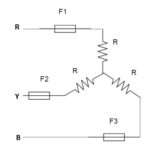h Parameter or Hybrid Parameter of Two Port Network

h parameter of two port network is a square matrix of order 2×2. Basically it is a way to represent a two port network. It is also known as hybrid parameter. In this form of representation, voltage of input port and current of the output...

What is Power Factor? – Definition and Formula

Power factor is an important parameter for the calculation of active and reactive power in electrical circuit. It has significance only for AC circuit. For DC circuit, it is not considered for power calculation.Its value is unity for DC circuit and may vary in between...

Z Parameter of Two Port Network

Z parameter is a factor by which input voltage and current & output voltage and current of two port network is related with. For any two port network, input voltage V1 and output voltage V2 can be expressed in terms of input current I1 and...

Power Triangle

Power Triangle is a right angled triangle whose sides represent the active, reactive and apparent power. Base, Perpendicular and Hypogenous of this right angled triangle denotes the Active, Reactive and Apparent power respectively. This triangle is not a new concept but it is just the...

Active, Reactive and Apparent Power

Active Power: Active power is the real power consumed in an electrical circuit. It is the useful power which can be converted into other form of energy like heat energy in heater, light energy in bulb etc. It is also known as true or real...

Final Value Theorem and Its Application

Final Value Theorem is used for determining the final value of a Laplace domain function F(s). Though we can always transform a time domain function into Laplace domain to apply Final Value Theorem. According to Final Value Theorem, final value of a function i.e. value...

Initial Value Theorem

Initial value Theorem is a very useful tool for transient analysis and calculating the initial value of a function f(t). This theorem is often abbreviated as IVT. The limiting value of a function in frequency domain when time tends to zero i.e. initial value can...

4 Most Asked Numerical QuestionsIn job interview often interviewer asks some numerical this is quit tricky and easy to answer without going into much calculation. In this post, I am going to discuss 3 most asked numerical questions in job interviews. These 3 questions are picked from my personal...